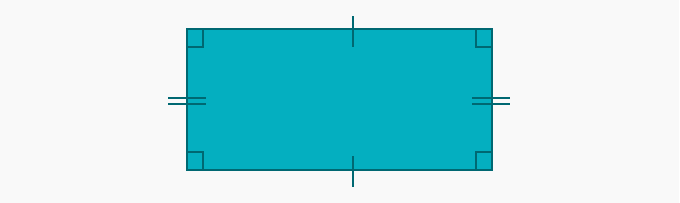Perimeter Of A Rectangle - Definition with Examples

The Complete K-5 Math Learning Program Built for Your Child

• 30 Million Kids

Loved by kids and parent worldwide

• 50,000 Schools

Trusted by teachers across schools

• Comprehensive Curriculum

Aligned to Common Core

Perimeter of a Rectangle

Rectangles are four-sided polygons. Following are the properties of a rectangle:

(i) All the angles of a rectangle are 90º.

(ii) Opposite sides of a rectangle are always the same in size.Perimeter of a rectangle

The perimeter of a rectangle is the total length of all the sides of the rectangle. Hence, we can find the perimeter by adding all four sides of a rectangle.Perimeter of the given rectangle is a + b + a + b. Since opposite sides of a rectangle are always equal, we need to find the dimensions of only two sides to find the perimeter of a rectangle.

The perimeter of the above rectangle with sides ‘a units’ and ‘b units’ is:

a + b + a + b = 2a + 2b = 2  (a + b) units.

Hence, the formula for the perimeter of a rectangle = 2 × (sum of adjacent sides)

Examples of finding the perimeter of a rectangle

Example 1. The two sides of the rectangle are given. What will be the perimeter of the rectangle?Solution: One side of the rectangle is 2 cm and the other side is 5 cm.

We know that, the perimeter of a rectangle = 2 × (sum of adjacent sides)

Therefore, the perimeter of the rectangle = 2 × (5 + 2) = 2 × (7) = 14 cm

Example 2. A rectangular playground is 20 m long and 13 m wide. Find its perimeter.Solution: One side of the rectangular playground is 20 m and the other side is 13 m.

We know that, the perimeter of a rectangle = 2 × (sum of adjacent sides)

Therefore, the perimeter of the rectangular ground = 2 × (20 + 13) = 2 × (33) = 66 m

Tricky problems involving perimeter of rectangles

Type I: When the perimeter and only one of the sides are given.

Example 1. If the perimeter of the given rectangle is 10 cm and the length of one of its sides is 2 cm. What will be the other side?Solution: The perimeter of the rectangle, with one of the sides equal to 2 cm, is 10 cm.

Let the missing side be ‘a’.

We know that, the perimeter of a rectangle = 2 × (sum of adjacent sides)

10 = 2 × (2 + a) 5 = (2 + a)

a = 5 - 2 = 3 cm

Type II: Finding sides using the properties of a rectangle.

Example 2. In the given rectangle, if  a = 4 cm and d = 3 cm. Find b and c.Solution: We know  that side a = 4 cm and side d = 3 cm.

To find side b and c, we use the property that the opposite sides of a rectangle are always the same in size.Hence, a = c = 4 cm and d = b = 3 cm.

 Fun Facts Many historical buildings have rectangle like shapes in them e.g. Parthenon in Athens.

Won Numerous Awards & Honors